# DETAIL EVERY ANSWER WITH THE EXCEL FORMULA TO SOLVE THE EQUATION - EVERY ANSWER NEEDS AN EXCEL EQUATION DETAIL EVERY ANS...

DETAIL EVERY ANSWER WITH THE EXCEL FORMULA TO SOLVE THE EQUATION - EVERY ANSWER NEEDS AN EXCEL EQUATION

DETAIL EVERY ANSWER WITH THE EXCEL FORMULA TO SOLVE THE EQUATION - EVERY ANSWER NEEDS AN EXCEL EQUATION

DETAIL EVERY ANSWER WITH THE EXCEL FORMULA TO SOLVE THE EQUATION - EVERY ANSWER NEEDS AN EXCEL EQUATION

DETAIL EVERY ANSWER WITH THE EXCEL FORMULA TO SOLVE THE EQUATION - EVERY ANSWER NEEDS AN EXCEL EQUATION

DETAIL EVERY ANSWER WITH THE EXCEL FORMULA TO SOLVE THE EQUATION - EVERY ANSWER NEEDS AN EXCEL EQUATION

DETAIL EVERY ANSWER WITH THE EXCEL FORMULA TO SOLVE THE EQUATION - EVERY ANSWER NEEDS AN EXCEL EQUATION

DETAIL EVERY ANSWER WITH THE EXCEL FORMULA TO SOLVE THE EQUATION - EVERY ANSWER NEEDS AN EXCEL EQUATION

DETAIL EVERY ANSWER WITH THE EXCEL FORMULA TO SOLVE THE EQUATION - EVERY ANSWER NEEDS AN EXCEL EQUATION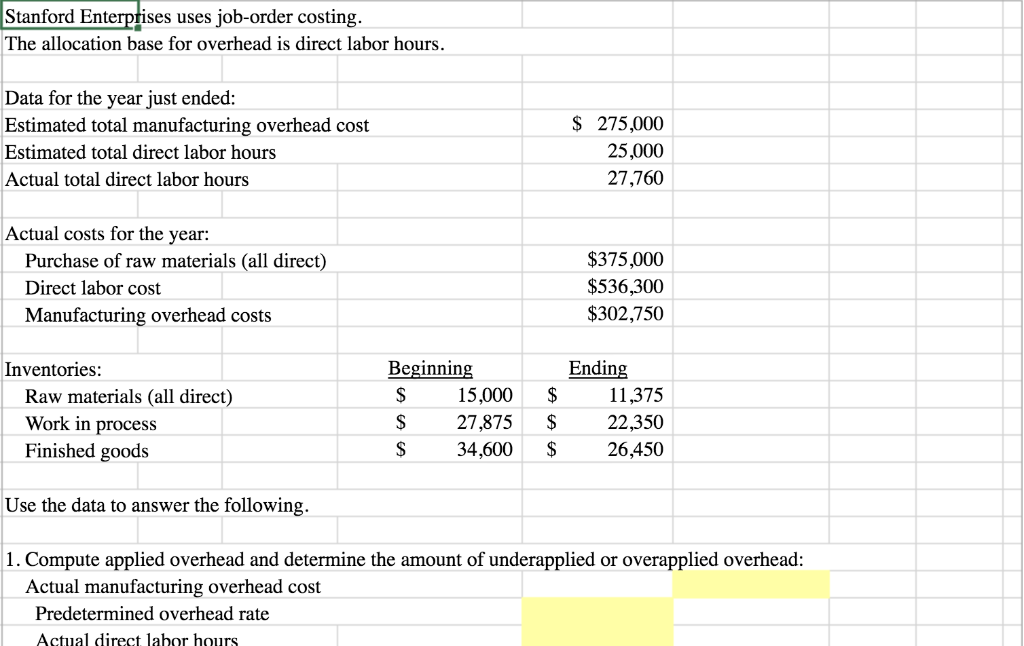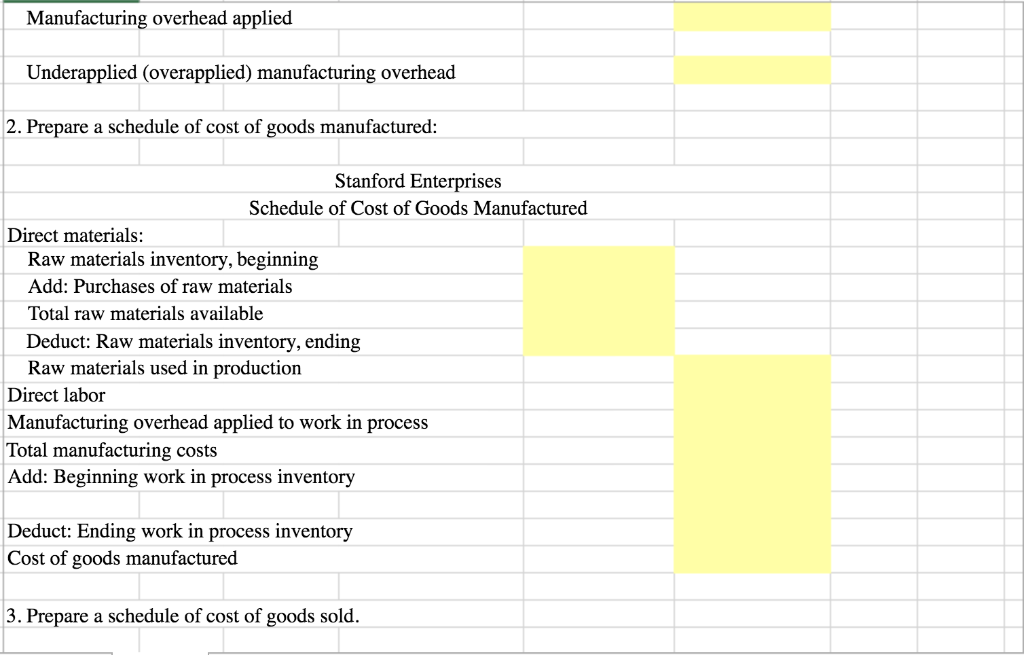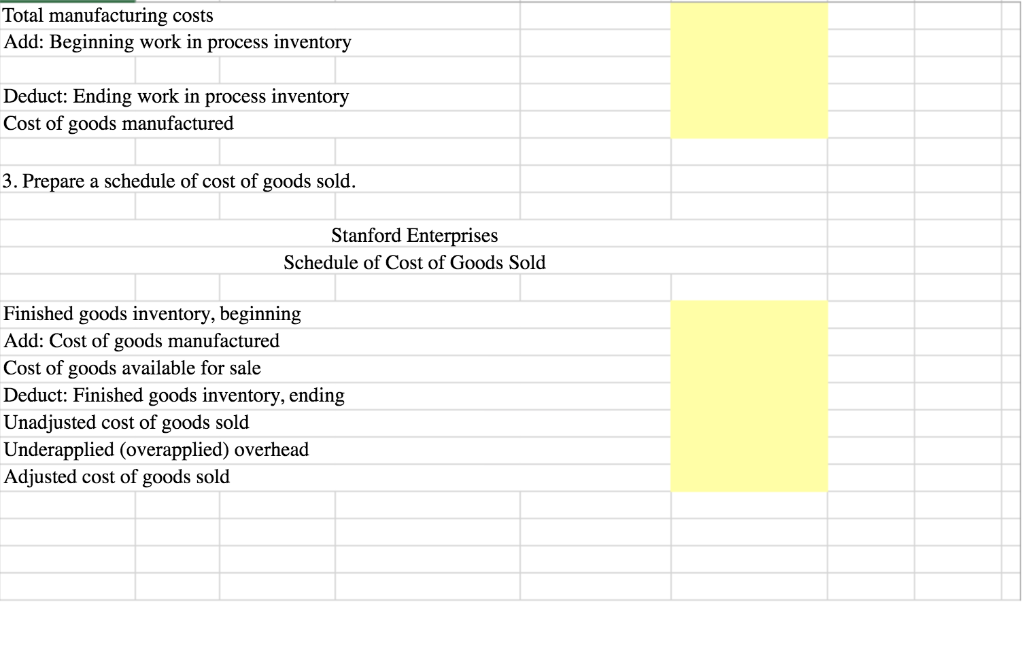Stanford Enterprises has provided its manufacturing estimated and actual data for the year end. The Controller has asked you to compute the predetermined overhead rate, the schedule of cost of goods manufactured, and the schedule of cost of goods sold. Use the information included in the Excel Simulation and the Excel functions described below to complete the task.

• Cell Reference: Allows you to refer to data from another cell in the worksheet. From the Excel Simulation below, if in a blank cell, “=E5” was entered, the formula would output the result from cell E5, or \$275,000 in this example.
• Basic Math functions: Allows you to use the basic math symbols to perform mathematical functions. You can use the following keys: + (plus sign to add), - (minus sign to subtract), * (asterisk sign to multiply), and / (forward slash to divide). From the Excel Simulation below, if in a blank cell “=E6+E7” was entered, the formula would add the values from those cells and output the result, or 52,760 in this example. If using the other math symbols the result would output an appropriate answer for its function.
• SUM function:  Allows you to refer to multiple cells and adds all the values. You can add individual cell references or ranges to utilize this function. From the Excel Simulation below, if in a blank cell “=SUM(E10,E11,E12)” was entered, the formula would output the result of adding those three separate cells, or 1,214,050 in this example. Similarly, if in a blank cell “=SUM(E10:E12)” was entered, the formula would output the same result of adding those cells, except they are expressed as a range in the formula, and the result would be 1,214,050 in this example.
Stanford Enterptises The allocation base for overhead is direct labor hours uses job-order costing Data for the year just ended: 275,000 Estimated total manufacturing overhead cost 25,000 Estimated total direct labor hours 27,760 Actual total direct labor hours Actual costs for the year: \$375,000 Purchase of raw materials (all direct) \$536,300 Direct labor cost \$302,750 Manufacturing overhead costs Beginning Ending Inventories: \$ 15,000 \$ 11,375 Raw materials (all direct) Work in process S \$ 22,350 27,875 Finished goods S \$ 34,600 26,450 Use the data to answer the following. 1. Compute applied overhead and determine the amount of underapplied or overapplied overhead: Actual manufacturing overhead cost Predetermined overhead rate Actual direct labor hours
Manufacturing overhead applied Underapplied (overapplied) manufacturing overhead 2. Prepare a schedule of cost of goods manufactured: Stanford Enterprises Schedule of Cost of Goods Manufactured Direct materials: Raw materials inventory, beginning Add: Purchases of raw materials Total raw materials available Deduct: Raw materials inventory, ending Raw materials used in production Direct labor Manufacturing overhead applied to work in process Total manufacturing costs Add: Beginning work in process inventory Deduct: Ending work in process inventory Cost of goods manufactured |3. Prepare a schedule of cost of goods sold
Total manufacturing costs Add: Beginning work in process inventory Deduct: Ending work in process inventory Cost of goods manufactured 3. Prepare a schedule of cost of goods sold. Stanford Enterprises Schedule of Cost of Goods Sold Finished goods inventory, beginning Add: Cost of goods manufactured Cost of goods available for sale Deduct: Finished goods inventory, ending Unadjusted cost of goods sold Underapplied (overapplied) overhead Adjusted cost of goods sold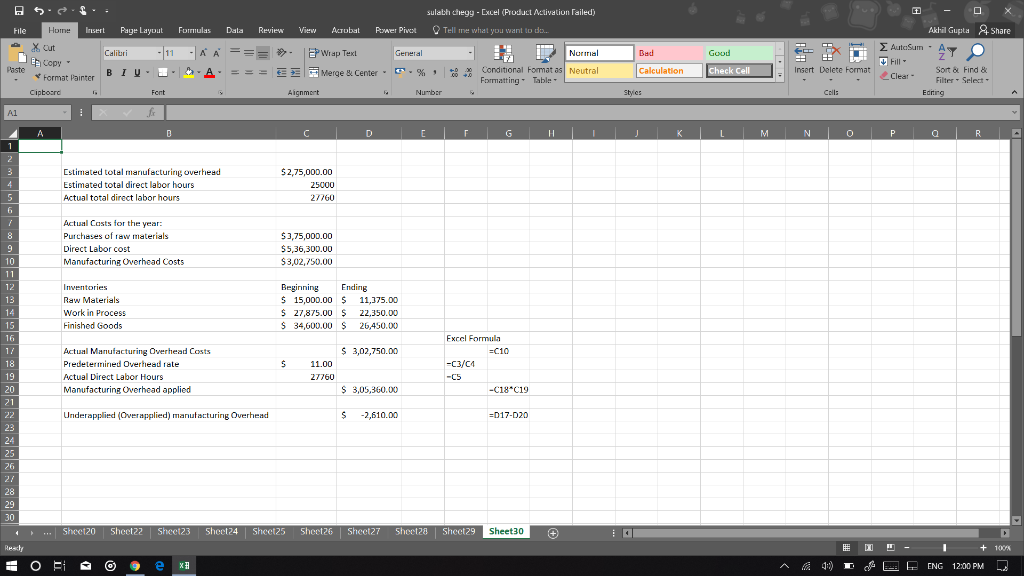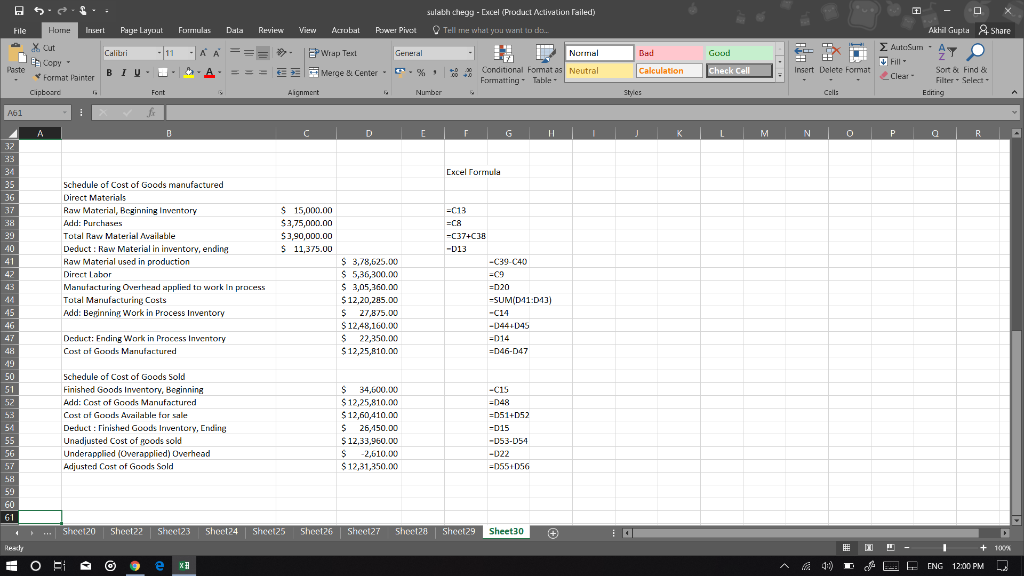##### Add Answer of: DETAIL EVERY ANSWER WITH THE EXCEL FORMULA TO SOLVE THE EQUATION - EVERY ANSWER NEEDS AN EXCEL EQUATION DETAIL EVERY ANS...
More Homework Help Questions Additional questions in this topic.